Total of questions: 284

 First steps (21 questions) Basic Settings (12 questions) Cells 1 (25 questions) Cells 2 (24 questions) WorkSheets (10 questions)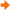Formulas and Functions (41 questions) Formatting (29 questions) Charts (24 questions) Output: Setup and Print (21 questions) Functions (14 questions) Images (3 questions) MS Excel 2016, 2013, 2010, 2007, 2003 (BENCHMARK) (30 questions) MS Excel 2016, 2013, 2010, 2007, 2003 (ASSESSMENT) (30 questions)

 1106 Enter a function in the cell C9 of the SALES worksheet in order to calculate the sum of the cell range C5:C8.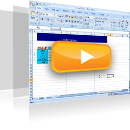1107 In the cell L5 of the SALES worksheet enter a formula that returns the quotient of the cell K5 divided by the cell K9 keeping the reference to the cell K9 stable. Extend the formula to the cell range L6:L9.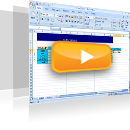1108 Enter a formula in the cell C10 that subtracts the value of the cell C5 from the value of the cell C9.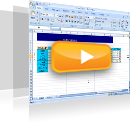1109 Enter the appropriate function in the cell C14 so as to calculate the maximum sales figure for January (cell range C5:C8).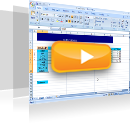1110 In the cell C15 enter a function which returns the word DELLA, if the content of the cell C5 is equal to the content of the cell C14; otherwise the formula returns the word other.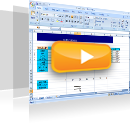1130 Correct the error of the formula in cell D19 of the 2ndhour2 worksheet.1132 Change the formula in the cell D27 of the SALES worksheet, so that if it is copied to the cell range D27:K31, it calculates correctly the hypothetical turnover for each way of payment. Apply the formula to this cell range.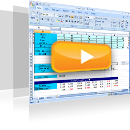1140 Rename the sheets of the active workbook as follows: Sheet1: Income Sheet2: Expenses Sheet3: Total Insert the value 1000 in the cell A1 of the Income worksheet. Insert the value 500 in the cell A1 of the Expenses sheet. Display the difference between the value of the cell A1 of the Income worksheet and the value of the cell A1 of the Expenses worksheet (NOT 1000-500), in the cell A1 of the Total worksheet. If the value of the cell A1 in the Income or in the Expenses worksheet changes, the value displayed on the Total worksheet should change as well.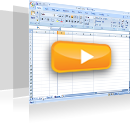1189 Given that sales equal to beginning pieces + supplies - end pieces - destroyed pieces, enter a formula in the cell range F7:F9 that returns the sales for each product. Enter a formula in the cell range H7:H9 that returns the sales value in Drachmas and use the result to calculate the sales value in Euros in the cell range I7:I9. (the euro-drachma exchange rate is provided in the cell G2)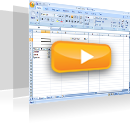1191 Reproduce the function of the cell C9 to the cell range D9:K9 of the SALES worksheet.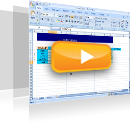1192 Change the function in cell B4, so that the reference to the cell A1 uses an absolute reference only by column.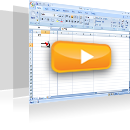1193 Change the function in the cell D2, so that the reference to the cell A1 is absolute only by row.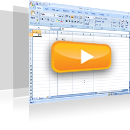1200 In the cell A1 enter a function that counts non blank cells for the cell range A3:A15000. Also, in the cell C116 enter a function that returns the number of cells that contain numbers for the cell range C3:C110 and in the cell C117 enter another function to return non blank cells for the same cell range.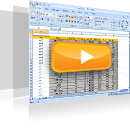1201 In the cell E116 enter a function that calculates the average cost of the products displayed in the cell range Å3:Å110.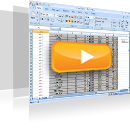1202 In the cell E116 enter a function that calculates the minimum cost for the cell range E3:E110 and in the cell E117 another function that returns the maximum cost for the same cell range.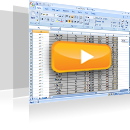1217 Enter the appropriate function in the cell B1, so that if the value in the cell (A1) is higher than 5000, the function will return 1. If the value in the cell A1 is less than or equal to 5000, the function will return 0. Reproduce the function up to the cell B10.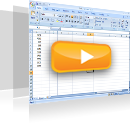1218 Insert the appropriate function in the cell E1, so that if the value in the cell A1 is higher than the value in the cell B1, the function will return the value of the cell C1; otherwise the function will return the value of the cell D1. Reproduce this function up to the cell E10.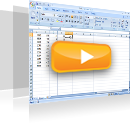1235 There is a message for an error in the cell G6. The formula doesn’t contain any errors. Without changing the cell G6, find out which is the cell to cause the error and insert the appropriate formula or function in it.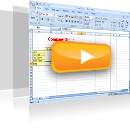1266 Make sure the indication #### in cell D7 is not displayed.1271 Automatically fill in the cell range A2:A1000 using the text of the cell A1.1297 The cell G9 displays the cost of the RAM memory in euros. Calculate the cost of the RAM memory in dollars in the cell H9 taking into account the euro to dollar exchange rate displayed in the cell E19. Then reproduce the formula in the cell range H10:H17.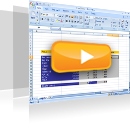1308 Enter in the cell E116 a function to calculate the minimum value of the cell range Å3:Å110.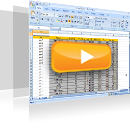1310 Insert in the cell C116 a function to count the total of cells containing numbers within the cell range C3:C110.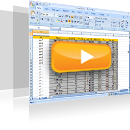1311 Insert a function in the cell A1 to count non blank cells within the cell range A3:A15000.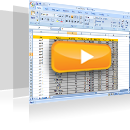1543 Insert the appropriate function in the cell range B1:B50 that rounds the values of column A to 4 decimal places. Insert the appropriate function in the cell range C1:C50 that rounds the values of column A to 2 decimal places. Insert the appropriate function in the cell range D1:D50 that rounds the values of column A to the left of the decimal point.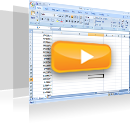1671 Use the appropriate function in the cell G12 to calculate the average of the values displayed in the cell range G5:G10.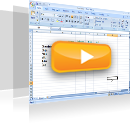1679 Use the appropriate function in the cell J4 to calculate the average number of items sold in April.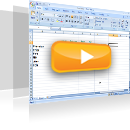1711 Use the appropriate function in the cell J4 to display the lowest value in the cell range D5:D10.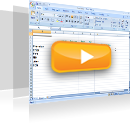1712 Navigate to the cell D12 and calculate the maximum value of the cell range D5:G10.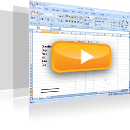1714 Navigate to the cell J4 and insert there the appropriate function to display the maximum value of the cell range F5:F10.1718 Navigate to the cell G13 and insert there the appropriate function to display the sum of the values appearing in the cell range G4:G10. Then, save changes.1750 Navigate to the cell H17 of the active worksheet and use the appropriate function to display the total of the cell range H6:H9.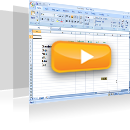1758 Navigate to the cell E11 of the SALES worksheet and insert there the appropriate function to display the sum of the values of the cell range E6:E9.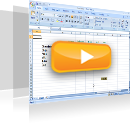1768 Navigate to the cell C14 of the active worksheet and insert there the appropriate function to display the average of the values of the cell range D6:D9.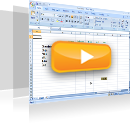1844 Use the appropriate function in the cell E11, to return the day of the date displayed in the cell E4.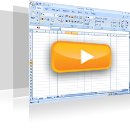1845 Use the appropriate function in the cell E14 to round the contents of the cell E11 to two decimal places.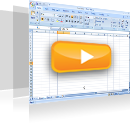1880 Use the proper function in the cell B1 to count the blank cells in the cell range A1:A400.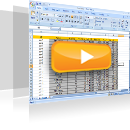10130 Match the error codes with their description.11044 The correct way to enter a formula is displayed in cell…12841 In order to apply an absolute reference only by column in cell B15, which of the following formulas is the correct one?12842 Which of the following formulas is the correct one in order to apply the correct absolute reference?